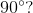# ISEE Lower Level Quantitative : Shape Properties

## Example Questions

1 3 Next →

### Example Question #21 : Shape Properties

Which shape has two sets of opposite, equal angles?

Kite

Trapezoid

Rhombus

Rhombus

Explanation:

A rhombas has two sets of opposite, equal angles.

A kite has to one one set of opposite, equal angles.

A quadrilateral and trapezoid do not have to have any equal angles.

### Example Question #600 : Plane Geometry

Which shape has to have all angles that equalSquare

Rhombus

Parallelogram

Kite

Square

Explanation:

A square has angles that all measureA kite, parallelogram, and rhombus do not have to have angles that measure### Example Question #1 : Classify Two Dimensional Figures: Ccss.Math.Content.5.G.B.4

Which shape has to have all angles that equalParallelogram

Rhobmus

Rectangle

Kite

Rectangle

Explanation:

A rectangle has angles that all measureA kite, parallelogram, and rhombus do not have to have angles that measure### Example Question #1 : Classify Two Dimensional Figures: Ccss.Math.Content.5.G.B.4

Which shape has to haveequal sides?

Rectangle

Parallelogram

Square

Kite

Square

Explanation:

A square has to haveequal sides.

A rectangle and parallelogram have opposite, equal side lengths.

A kite has two pairs of adjacent sides that are equal.

### Example Question #22 : Shape Properties

What two shapes have to have two sets of opposite angles that are equal in measure?

Rhombus and Kite

Parallelogram and Rhombus

Parallelogram and Kite